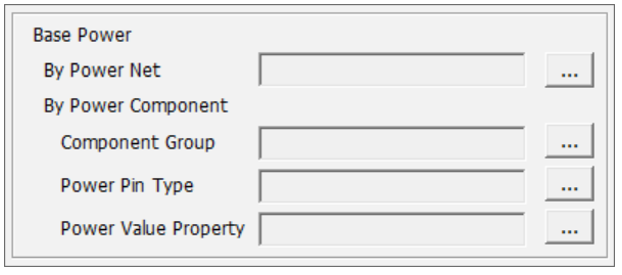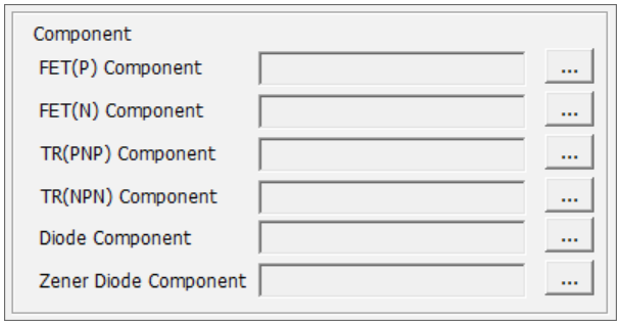# Power Rail Group Type Setup

To perform a Stress Test, the voltage value of each power and ground nets should be defined.

You can define voltage values of each power net and voltage of voltage divide logic.

• Power Net Group: Select power and ground net group to define voltage value.
• Net: Upon selecting this menu, all the selected power and ground nets are listed in the table below.
• Group: Upon selecting this menu, all the selected power and ground groups are listed in the table below.
• Find Voltage: Upon clicking this button, the voltage value of power and ground nets are displayed in the table below. To execute this menu, the table below must be setup first.

There are three types: Pin Property, Net Name, and Voltage types.

• Pin Property: You can assign power value using pin property of component which is connected to each power net.
• Net Name: If the power net name contains a voltage value information, you can assign power value using net name string of power net.
• Voltage(V): You can enter voltage value of power net.
After selecting the Type field to Pin Property and click the empty raw of Property field, the Select Pin Property dialog opens.

## Power Rail Group Setup

1. Power Net Group: Select power and ground net group to define voltage value.
2. Net: Upon selecting this menu, all the selected power and ground nets are listed in the table below.
3. Group: Upon selecting this menu, all the selected power and ground groups are listed in the table below.
4. Select a Type and follow the steps for each:
• To assign power value using pin property of component which is connected to each power net, select Pin Property.
1. Click the empty Property field to open the Select Pin Property dialog.
2. Select the appropriate property, and then click OK.
• To assign power value using net name string of power net, select Net Name
1. Enter the voltage separator in the Net Name Filter field.
Note: The voltage separator means that the character used to distinguish the decimal point of voltage. Ex: VCC1P8 => the voltage separator is P Ex: VCC1.8 => the voltage separator is “.” In this case, enter “.,P” as a voltage separator.
• To enter your own voltage value, select Voltage(V).
1. Enter the voltage value directly in the Voltage(V) filed.
5. Find Voltage: Upon clicking this button, the voltage value of power and ground nets are displayed in the table below.

## Voltage Divide Logic

You can find out the exact voltage value of voltage divide logic.

1. Upon clicking Voltage Divide Logic, the Voltage Divide dialog opens.
2. Voltage Divide Component Section: In this step, we set up the component list used for voltage divide logic and property in which the resistance value is declared.
1. Component: Select component group used for voltage divide logic.
2. Component Value: Select property in which the resistance value is declared.
3. Power Composite Component Section: In this step, we declare the component to be processed short and the component to be opened during power trace navigation.
Note: Since Stress Test is a DC level test, the capacitor is considered as open and inductor is considered as short. Transistor or FET is assumed to be turned on and treated as either short or considering turn-on voltage. And TR or FET is a device with three pins, so it needs information about pins connected to each other during short processing.
1. Short Component: Select the component group to be processed short during power trace navigation.
2. Open Component: Select the component group to be processed open during power trace navigation.
4. Short Pin Section: TR or FET is a device with three pins, so it needs information about pins connected to each other during short processing.
1. Short Pin-By Pin Name: Declare pin paring information as pin name. If there is more than one condition, the separator “,” is used.
2. Short Pin-By Signal Name: Declare pin paring information as signal name. If there is more than one condition, the separator “,” is used.
5. Consider Turn-on Voltage-Base Power Section: In this step, we declare the base power net to start navigation, and declare the power component used for power logic.
1. Consider Turn-on Voltage: Upon selecting this option, consider turn-on voltage during power navigation.2. By Power Net: Select base power net to start navigation.
3. By Power Component: Declare base power net to start navigation using component. Among the power nets, power nets that connected to specific pin of specific component are selected as base power net.
• Component Group: Select Component Group. Normally, the primary power comes from a power component such as DCDC converter.
• Power Pin Type: Select Power Pin Type. Among the power nets, power nets that connected to this type of pin are selected as base power net.
• Power Value Property: Select property in which the power value is declared.
6. Consider Turn-on Voltage-Component Section:1. Component: Declare the power component used for power logic from the following:
• FET(P) Component: Select P_channel FET component Group.
• FET(N) Component: Select N_channel FET component Group.
• TR(PNP) Component: Select PNP type Transistor component Group.
• TR(NPN) Component: Select NPN type Transistor component Group.
• Diode Component: Select Diode component Group.
• Zener Diode Component: Select Zener Diode component Group.
7. Turn-on Voltage Section: In this step, we declare the turn-voltage of each component group to be used during power navigation.
1. Turn-on Voltage: Select
2. Comp Group: Select component group with turn-on characteristic.
3. Voltage(V): Enter turn-voltage directly or select property in which the turn-on voltage is declared.
8. Show Voltage Divide Data & Voltage Assigned Data Section: In this step, you can review the voltage of voltage divide logic.
1. Show Voltage Divide Data & Voltage Assigned Data: Upon clicking this button, the voltage of each voltage divide logic will be displayed.